#### LET，一个超强大Excel新函数即将问世！

Excel精英培训 2020-09-17 09:07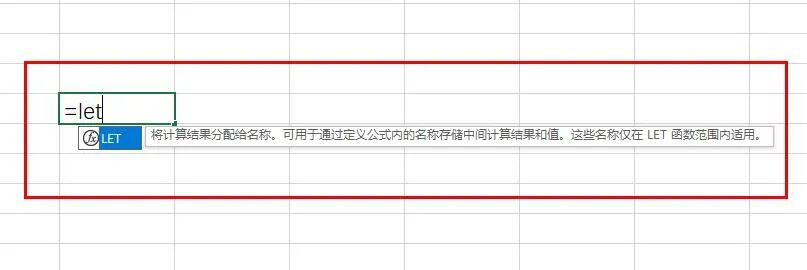LET 函数会向计算结果分配名称。这样就可存储中间计算、值或定义公式中的名称。这些名称仅可在 LET 函数范围内使用。与编程中的变量类似，LET 是通过 Excel 的本机公式语法实现的。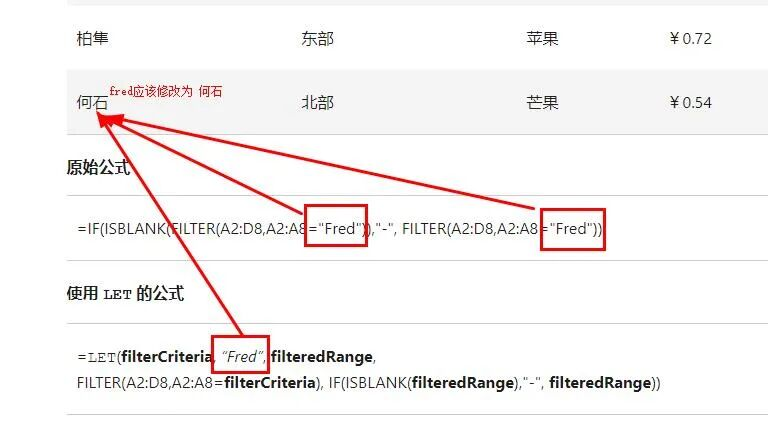3^3+6^2+6)^3+（3^3+6^2+6)^2+（3^3+6^2+6)*100

x=（3^3+6^2+6)

x^3+x^2+x*100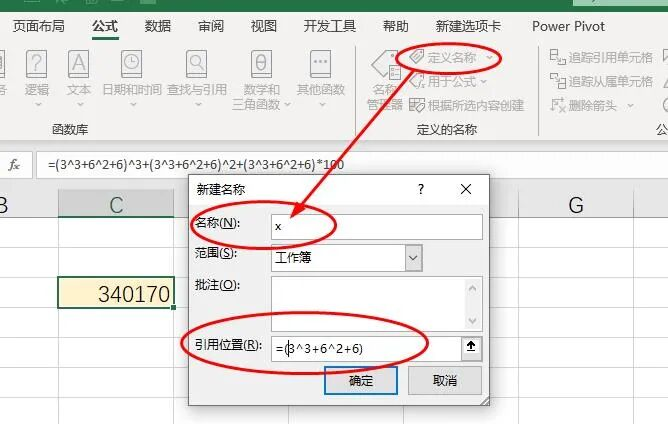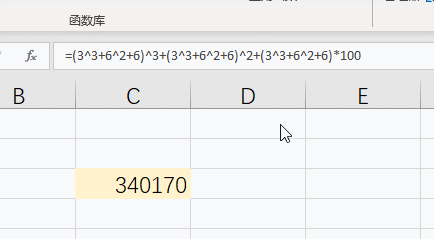=LET(x,3^3+6^2+6,x^3+x^2+x*100)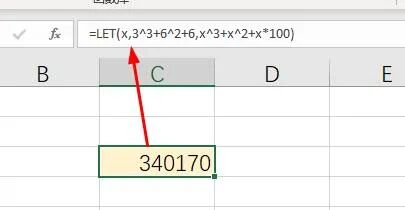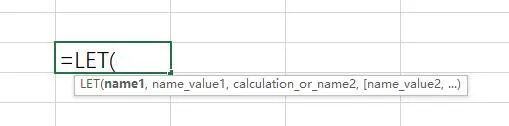=LET(名称1,名称1的表达式或值,名称2,名称2的表达式或值,....,含名称运算式）

let不只定义一个名称，它可以定义最多126个名称，在公式中名称和名称的表达式是成对出现的。

=let(2x+10)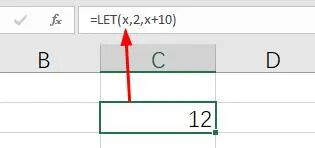=LET(x,2,y,5,x*y)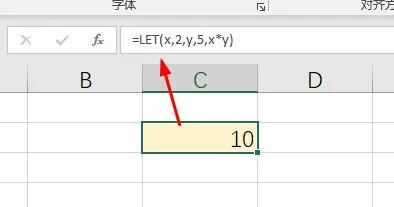【例】如下图所示为销量表，要求根据名称从销售中找到销售并按以下规则计算提成：

• 如果销量大于50，则销量*3

• 如果销量大小100，则销量*5

• 如果销量小于等于50，则销量*2

=IF(VLOOKUP(D2,A:B,2,0)>50,VLOOKUP(D2,A:B,2,0)*3,IF(VLOOKUP(D2,A:B,2,0)>100,VLOOKUP(D2,A:B,2,0)*5,VLOOKUP(D2,A:B,2,0)*2))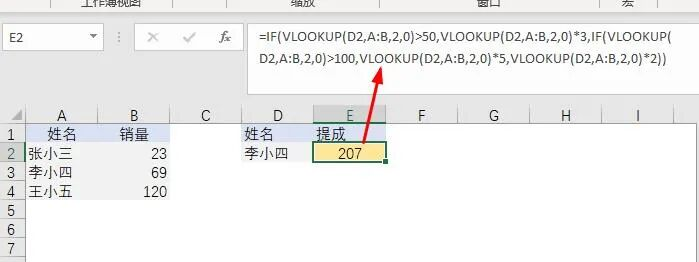=LET(x,VLOOKUP(D2,A:B,2,0),IF(x>50,x*3,IF(x>100,x*5,x*2)))